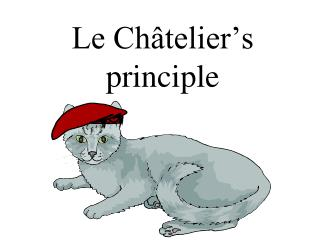DownloadDownload PresentationLe Châtelier’s principle

# Le Châtelier’s principle

Télécharger la présentation## Le Châtelier’s principle

- - - - - - - - - - - - - - - - - - - - - - - - - - - E N D - - - - - - - - - - - - - - - - - - - - - - - - - - -
##### Presentation Transcript

1. Le Châtelier’s principle

2. Kc = The significance of Kc values If Kc is small (0.001 or lower), [products] must be small, thus forward reaction is weak If Kc is large (1000 or more), [products] must be large, thus forward reaction is strong If Kc is about 1, then reactants and products are about equal but not exactly since they may be raise to different exponents Reactants  Products [Products] [Reactants]

3. Stresses to equilibria • Changes in reactant or product concentrations is one type of “stress” on an equilibrium • Other stresses are temperature, and pressure.

4. The response of equilibria to these stresses is explained by Le Chatelier’s principle: If an equilibrium in a system is upset, the system will tend to react in a direction that will reestablish equilibrium • Thus we have: 1) Equilibrium, 2) Disturbance of equilibrium, 3) Shift to restore equilibrium • Le Chatelier’s principle predicts how an equilibrium will shift (

5. Summary of Le Chatelier’s principle • Amounts of products and reactants: equilibrium shifts to compensate • N2  H2 E.g. N2 + 3H2 2 NH3 + 92 kJ N2 + 3H2 2NH3 + 92 kJ shift right N2 + 3H2 2NH3 + 92 kJ N2 + 3H2 2NH3 + 92 kJ N2 + 3H2 2NH3 + 92 kJ shift left N2 + 3H2 2NH3 + 92 kJ N2 + 3H2 2NH3 + 92 kJ Temperature: equilibrium shifts to compensate:  Heat N2 + 3H2 2NH3 + 92 kJ shift left N2 + 3H2 2NH3 + 92 kJ N2 + 3H2 2NH3 + 92 kJ Pressure (due to decreased volume): increase in pressure favors side with fewer molecules

6. Part 1: changes in reactant or product concentrations • Cu+2 + 4NH3 Cu(NH3)4+2 +4H2O • Ni+2 + 4NH3 Ni(NH3)6+2 +6H2O NH3+ H+ (NH4)

7. Part A: copper and nickel ions • Do not do Nickel ions • Question 4 the answer is pale green • Question 5 the answer is Violet • Question 6 the answer is pale green

8. Part B: Cobalt ionsDo not do • Co+2 + 4Cl- CoCl4-2 +6H2O • Question 8 the answer is pale pink • Question 9 the answer is deep blue • Question 10 the answer is pale pink

9. Part II. Equilibria involving sparingly soluble salts • Ag+ + CO3-2 Ag2CO3-2 • 2H+ + CO3-2 H2O + CO2

10. Part II. Equilibria involving sparingly soluble salts • Ag+ + Cl-AgCl • Ag+ + 2NH3 Ag(NH3)2 + heat NH3+ H+ (NH4)

11. Part III. Temp on Equilibria • Heat + CoCl2 + 6H2O Co(H2O)6 + 4Cl